# 机器学习——逻辑回归(logistic regression)

##### 一、逻辑回归原理

Logistic回归虽然名字里带“回归”，但是它实际上是一种分类方法，主要用于两分类问题（即输出只有两种，分别代表两个类别）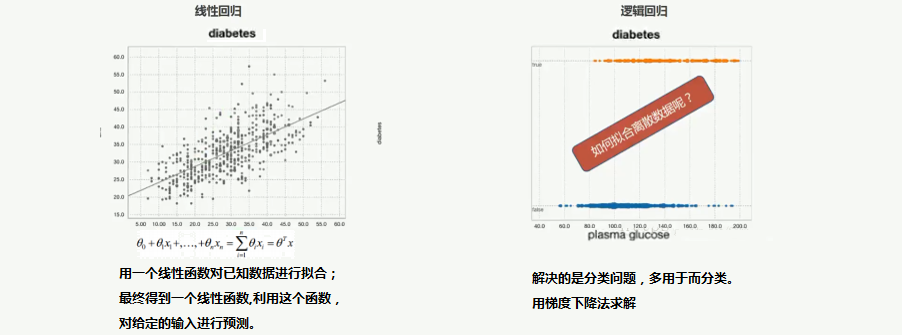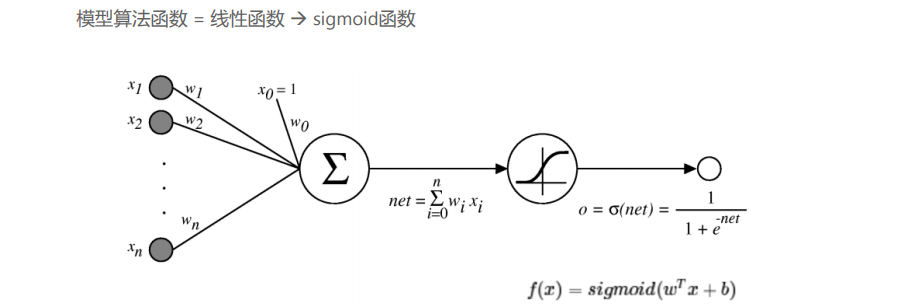P(x)=11+e(β0+β1x)       β0β1 P ( x ) = 1 1 + e − ( β 0 + β 1 x )               其 中 β 0 ： 截 距 。 β 1 ： 系 数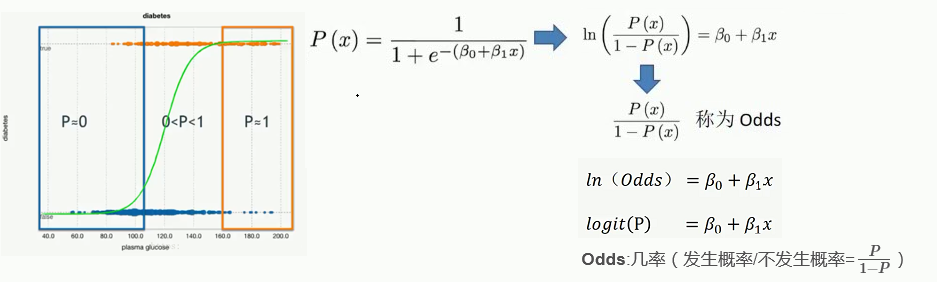##### 二、最大似然估计 正则化

Cos(y,β)={ln(P)ln(1P)y=1             P=1ln(P)=0y=0             P=0ln(P)= C o s ( y , β ) = { − l n ( P ) y = 1                           当 P = 1 ⇨ − l n ( P ) = 0 − l n ( 1 − P ) y = 0                           当 P = 0 ⇨ − l n ( P ) = ∞

J(β)=1mi=1mCos(y,β) J ( β ) = 1 m ∑ i = 1 m C o s ( y , β )

J(β)=1mi=1mCos(y,β)+λ2mj=1nβ2j J ( β ) = 1 m ∑ i = 1 m C o s ( y , β ) + λ 2 m ∑ j = 1 n β j 2

● 当模型参数 β 过多时，损失函数会很大，算法要努力减少 β 参数值来让损失函数最小。
● λ 正则项重要参数，λ 越大惩罚越厉害，模型越欠拟合，反之则倾向过拟合。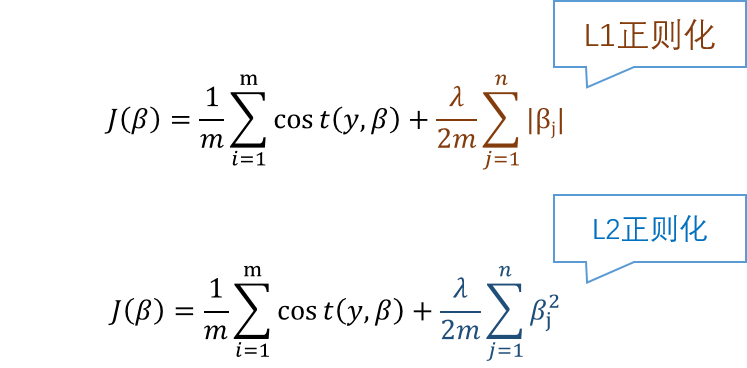● L1 正则化得到稀疏的权重值（可理解为去掉特征项权值）
● L2 正则化得到平滑的权值（可理解为减少特征项权重的比重）
##### 三、逻辑回归代码（结果解释）
Scikit-Learn(SkLearn)
http://scikit-learn.org/stable/index.html
代码：
from sklearn.linear_model import LogisticRegression  #逻辑回归
LogisticRegression(
penalty = '12',
dual = False,
tol = 0.0001,
C = 1.0,
fit_intercept = True,
intercept_scaling = 1,
class_weight = None,
random_state = None,
solver = 'liblinear',
max_iter = 100,
multi_class = 'ovr',
verbose = 0,
warm_state = False,
n_jobs = 1
)

penalty：（惩罚项） str,'l1'or'l2', default:'l2'-L1和L2正则化
C： float,default:  1.0--正则化强度的倒数，越小-->越强的正则化（惩罚越大）
fit_intercept:      bool,default:True--是否拟合截距
class_weight:       dict or 'balanced',default:None--是否对样本加权
solver:             {'newton-cg','lbfgs','liblinear','sag'},\
default:'liblinear' ---参数优化求解算法
multi_class:        str,{'ovr','multinomial'},default:'ovr' --多分类问题处理方法

Coeficient-系数
coef_:array,shape(n_classes,n_features)
Coeficient of the feature in the decision function
intercept_: array,shape(n_classes,)


Odd Ratio (比值 比)
In(Odds1/Odds0) = beta1

##### 四、模型自动化调参（多分类问题/特征变换）
在 Sklearn中用 GridSearchCV 自动化调参
from sklearn.grid_search import GridSearchCV
GridSearchCV(
esmtimator,
param_grid,
scoring = None,
fit_params = None,
n_jobs = 1,
iid = True,
refit = True,
cv = None,
verbose = 0
pre_dispatch = '2*n_jobs',
error_score = 'raise',
return_train_score = True
)
一些重要的参数：
esmtimator: esmtimator object --选择一个分类模型
param_grid: dict or list of dictionaries --待搜索的所有参数组合
scoring：--模型评估指标：accuracy，precision recall
cv： ---K折 cross validtion的K值
4.1 多分类问题处理

4.2 类别型特征变换
onehot 独热编码
4.3 连续型特征变换

4.4 特征组合

4.5 预测概率转换为分数

##### 五、准确率 和 召回率 （收益曲线 -ROC曲线和AUC ）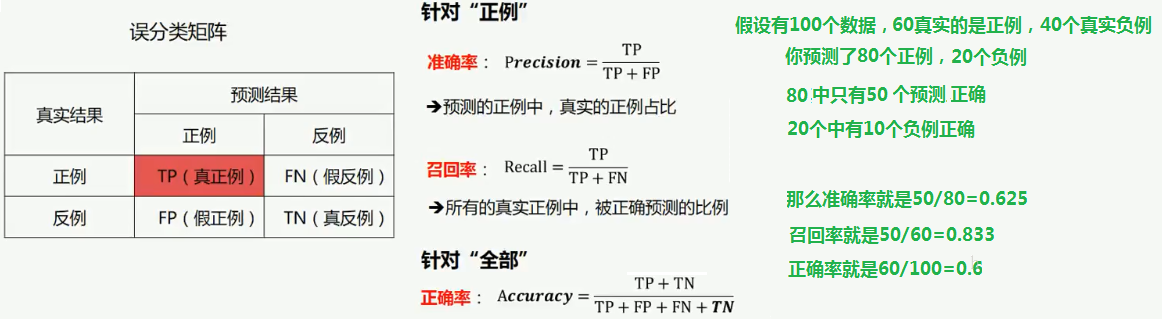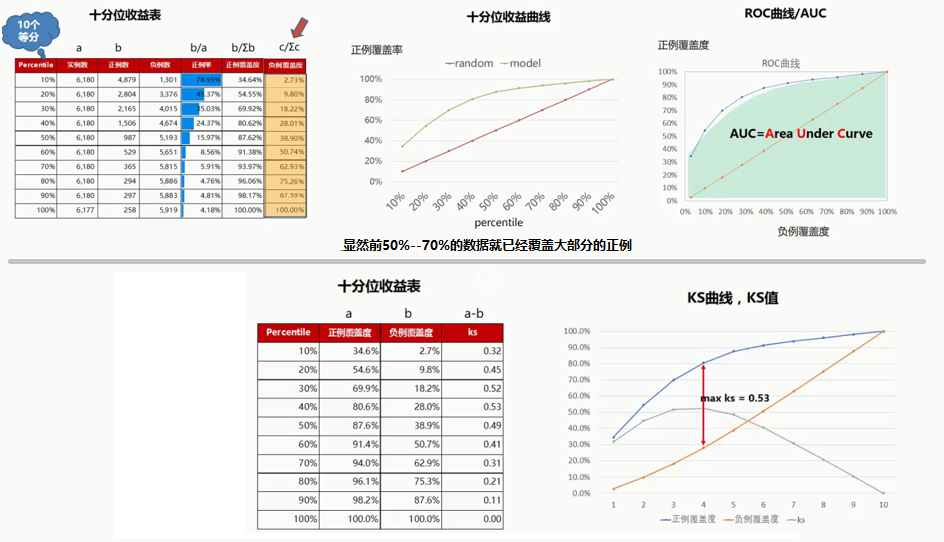##### 六、K折交叉验证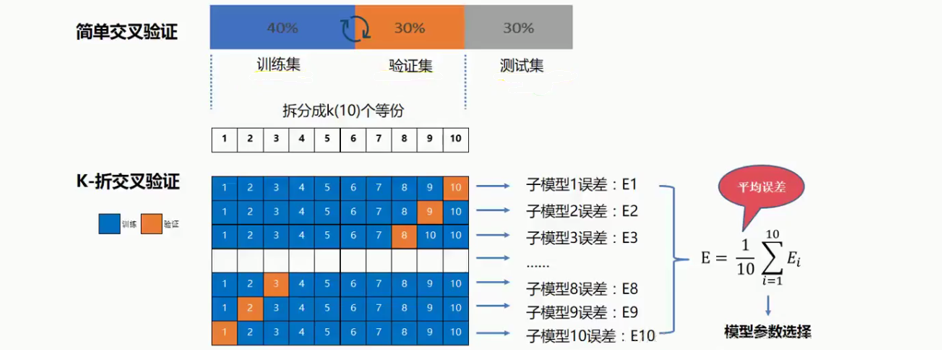11-1022万+04-091万+
03-024637
08-146万+
11-138万+
04-296093
10-141102
03-192万+
07-15369
08-164396
09-042854
04-291万+
08-1675
09-173220
11-244834
11-083006
02-142306
11-204722
©️2020 CSDN 皮肤主题: 1024 设计师:白松林